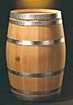Search IntMath
Close

450+ Math Lessons written by Math Professors and Teachers

5 Million+ Students Helped Each Year

1200+ Articles Written by Math Educators and Enthusiasts

Simplifying and Teaching Math for Over 23 Years

# Applications of Integration

by M. Bourne

## Chapter Contents

1. Applications of the Indefinite Integral shows how to find displacement (from velocity) and velocity (from acceleration) using the indefinite integral. There are also some electronics applications in this section.

In primary school, we learned how to find areas of shapes with straight sides (e.g. area of a triangle or rectangle). But how do you find areas when the sides are curved? We'll find out how in:4. Volume of Solid of Revolution explains how to use integration to find the volume of an object with curved sides, e.g. wine barrels.

5. Centroid of an Area means the centre of mass. We see how to use integration to find the centroid of an area with curved sides.

6. Moments of Inertia explains how to find the resistance of a rotating body. We use integration when the shape has curved sides.

7. Work by a Variable Force shows how to find the work done on an object when the force is not constant. This section includes Hooke's Law for springs.

### Survival Tips

Before you start this section, it's a good idea to revise:

(This chapter is easier if you can draw curves confidently.)

You may also wish to see the Introduction to Calculus.

8. Electric Charges have a force between them that varies depending on the amount of charge and the distance between the charges. We use integration to calculate the work done when charges are separated.

9. Average Value of a curve can be calculated using integration.

Head Injury Criterion is an application of average value and used in road safety research.

10. Force by Liquid Pressure varies depending on the shape of the object and its depth. We use integration to find the force.

In each case, we solve the problem by considering the simple case first. Usually this means the area or volume has straight sides. Then we extend the straight-sided case to consider curved sides. We need to use integration because we have curved sides and cannot use the simple formulas any more.

The chapter begins with 1. Applications of the Indefinite Integral »

## Problem SolverThis tool combines the power of mathematical computation engine that excels at solving mathematical formulas with the power of GPT large language models to parse and generate natural language. This creates math problem solver thats more accurate than ChatGPT, more flexible than a calculator, and faster answers than a human tutor. Learn More.